oxon88

## Homework Statement

A tubular column supports a mass as shown in the diagram below. A
strain gauge is placed transversely on the outside diameter of the bar to
act as a load measuring device.

The column has an outside diameter of 50 mm and an inside diameter of
40 mm. The modulus of elasticity of the tube material is 250 GN m–2 and
the poison’s ratio is 0.33.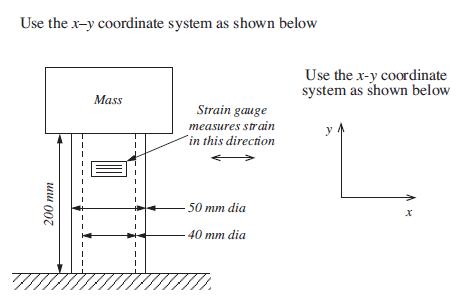Q. Sketch a graph of the expected strain against the applied load, for a
load range from 0 to 500 kg. Make load the horizontal axis on the
graph and strain the vertical axis.

## Homework Equations

Force,N = M.G

Area,A = ∏/4 . (Do2 - Di2) = ∏/4 (0.05m2) - (0.04m2) = 7.0685834 x10-4 m2

Normal Stress, σ = F / A

Normal Strain, ε = deformation in length / Original Length = l - l0 / l0

Young's Modulus, E = σ / ε = 250 GN m–2 = 250x10-9 N m-2

ε = σ / E

Poisson's Ration, V = 0.33

Transversal Strain, εt = -νε

## The Attempt at a Solution

Calculate Force, N, for range 0kg to 500kg using using F=MG

Calculate Normal Stress for the range 0kg to 500kg using σ = F/A

Calculate Normal Strain for the range 0kg to 500kg using ε = σ / E

Calculate Transverse Strain for the range 0kg to 500kg using εt = -νε

Plot a graph using Transverse Strain εt Vs Force, N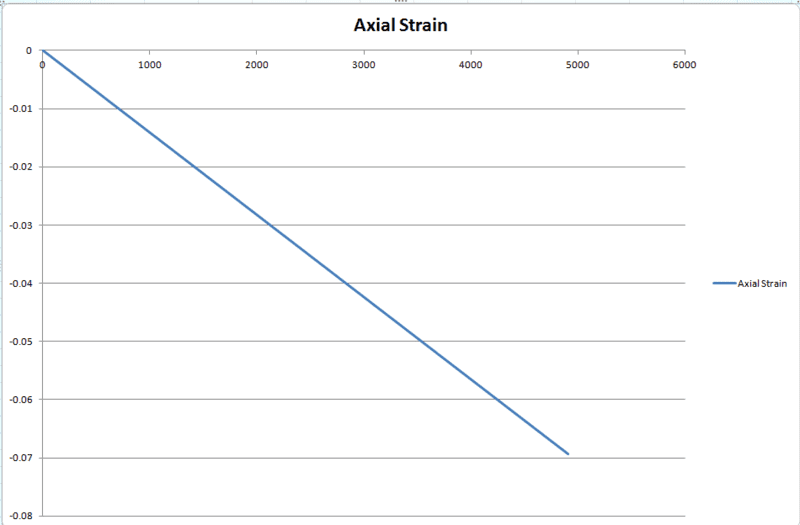Does this look anywhere near correct?

## Answers and Replies

oxon88
Anyone?

Staff Emeritus
Homework Helper
E should be 250*10^9 N/m^2

oxon88
ok so i had another go, changing the modulus of elasticity. The graph looks much the same as before.

Does this look correct?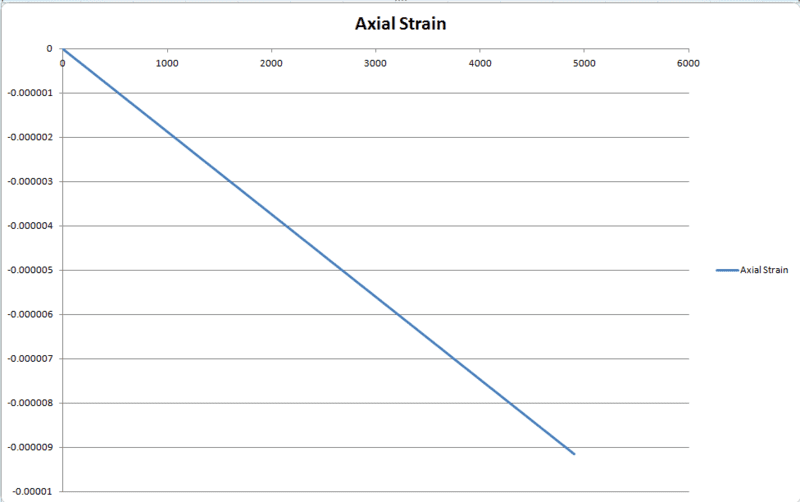Mentor
Please show us your numbers for 500 kg. Also, aren't you asked to plot the transverse strain, rather than the axial strain? Also, the axial strains are negative, and the transverse strains are positive.

oxon88
Load(Kg) | Load (N) | Normal Stress | Normal Strain | transverse strain | longitudinal strain

100kg | 981N | 1387831.118 | 5.55132E-06 | 1.83194E-06 | -5.55132E-06
200kg | 1962N | 2775662.235 | 1.11026E-05 | 3.66387E-06 | -1.11026E-05
300kg | 2943N | 4163493.353 | 1.6654E-05 | 5.49581E-06 | -1.6654E-05
400kg | 3924N | 5551324.47 | 2.22053E-05 | 7.32775E-06 | -2.22053E-05
500kg | 4905N | 6939155.588 | 2.77566E-05 | 9.15969E-06 | -2.77566E-05​

here are my numbers for 100Kg to 500Kg. apologies for the formatting.

oxon88
Graph looks like this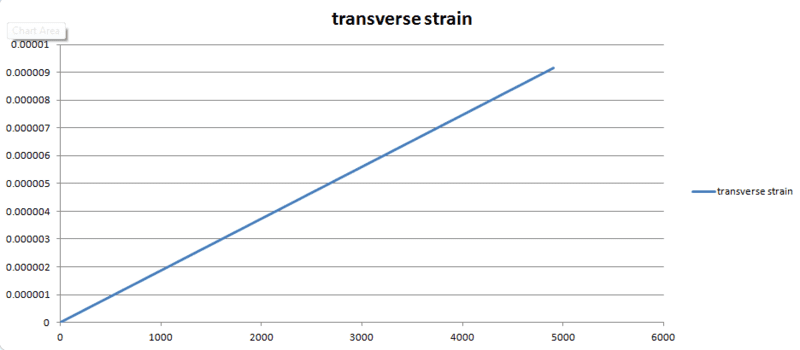Mentor
Load(Kg) | Load (N) | Normal Stress | Normal Strain | transverse strain | longitudinal strain

100kg | 981N | 1387831.118 | 5.55132E-06 | 1.83194E-06 | -5.55132E-06
200kg | 1962N | 2775662.235 | 1.11026E-05 | 3.66387E-06 | -1.11026E-05
300kg | 2943N | 4163493.353 | 1.6654E-05 | 5.49581E-06 | -1.6654E-05
400kg | 3924N | 5551324.47 | 2.22053E-05 | 7.32775E-06 | -2.22053E-05
500kg | 4905N | 6939155.588 | 2.77566E-05 | 9.15969E-06 | -2.77566E-05​

here are my numbers for 100Kg to 500Kg. apologies for the formatting.
I spot checked your numbers, and they look good. What sign convention do you use for stress; is compressive stress regarded as positive or negative?

oxon88
Stresses that result in extension, or tensile stresses, are defined as positive, and
compressive stresses as negative in sign.

Mentor
Stresses that result in extension, or tensile stresses, are defined as positive, and
compressive stresses as negative in sign.
If this is the case, then the normal stresses in your table should be negative, and the normal strains should be the same as the longitudinal strains.

oxon88
ok I've had a rethink and changed the table. I'm a little confused as I believe the transverse strains should be positive in value. however it is showing as negative.

Load(Kg) | Load (N) | Normal Stress | Normal Strain | transverse strain | longitudinal strain

-100 | -981 | -1387831.118 | -5.55132E-06 | -1.83194E-06 | -5.55132E-06
-200 | -1962 | -2775662.235 | -1.11026E-05 | -3.66387E-06 | -1.11026E-05
-300 | -2943 | -4163493.353 | -1.6654E-05 | -5.49581E-06 | -1.6654E-05
-400 | -3924 | -5551324.47 | -2.22053E-05 | -7.32775E-06 | -2.22053E-05
-500 | -4905 | -6939155.588 | -2.77566E-05 | -9.15969E-06 | -2.77566E-05

Last edited:
Mentor
The transverse strain should be opposite in sign to the longitudinal strain. If the cylinder compresses axially, it must expand in the radial and circumferential directions. That's what your Poisson Ratio equation says.

Chet

oxon88
ok. So i changed the signs. I now have these figures and the graph is below. Does this now look ok?

Load(Kg)| Load(N) | Normal Stress | Normal Strain | Transverse Strain | Longitudinal Strain
100 | 0981 | -1387831.118 | -5.55132E-06 | 1.83194E-06 | -5.55132E-06
200 | 1962 | -2775662.235 | -1.11026E-05 | 3.66387E-06 | -1.11026E-05
300 | 2943 | -4163493.353 | -1.66540E-05 | 5.49581E-06 | -1.6654E-05
400 | 3924 | -5551324.470 | -2.22053E-05 | 7.32775E-06 | -2.22053E-05
500 | 4905 | -6939155.588 | -2.77566E-05 | 9.15969E-06 | -2.77566E-05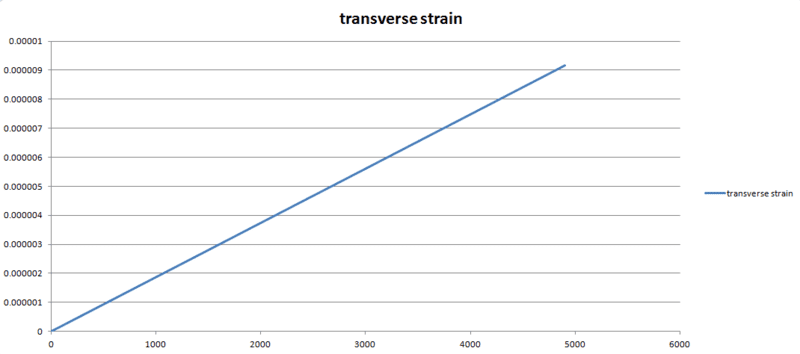Mentor
Looks good.

Chet

oxon88
Great Thank you. The next part to the question has me a bit confused.

Show with the aid of a diagram how the orientation of the strain gauge could be changed to provide a greater strain for the same load. Sketch a graph of strain against applied load when the strain gauge is in
the new position.

I'm thinking that maybe the strain gauge could be mounted at a 45° angle. But would this give greater strain for the same load? Or am I over thinking this and should it just be mounted vertically?

Mentor
Great Thank you. The next part to the question has me a bit confused.

Show with the aid of a diagram how the orientation of the strain gauge could be changed to provide a greater strain for the same load. Sketch a graph of strain against applied load when the strain gauge is in
the new position.

I'm thinking that maybe the strain gauge could be mounted at a 45° angle. But would this give greater strain for the same load? Or am I over thinking this and should it just be mounted vertically?
Have you learned how to express the strain in an arbitrary direction in terms of the components of strain in the principal directions? Is the question asking about the absolute magnitude of the strain in an arbitrary direction (i.e., irrespective of its sign) or about the actual strain in an arbitrary direction? I can see why you are confused.

oxon88
I have nothing in my learning material that details anything like that.

here is the question: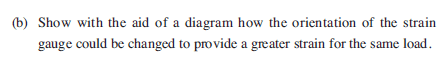Mentor
I have nothing in my learning material that details anything like that.

here is the question:

View attachment 62132
View attachment 62131
Well, OK, if you're talking about the absolute magnitude of the strain, and not whether it is extensional or compressive, then you can mount the strain gage in the vertical direction. That will give you the maximum absolute magnitude. However, if you're including the sign of the strain, the maximum value of strain is in the circumferential direction.

•1 person
oxon88
Many Thanks. You are a great help :)

oxon88
the next part of the question asks to Calculate the change in:
(i) the outside diameter (Do)
(ii) the inside diameter (Di)
(iii) the column length (l)

I have got the following answers:

(i) = 0.000457984 mm
(ii) = 0.000366387 mm
(iii) = -0.005551324 mm

do these answers look reasonable?

Mentor
the next part of the question asks to Calculate the change in:
(i) the outside diameter (Do)
(ii) the inside diameter (Di)
(iii) the column length (l)

I have got the following answers:

(i) = 0.000457984 mm
(ii) = 0.000366387 mm
(iii) = -0.005551324 mm

do these answers look reasonable?

I think these are correct for the data given.

Big Jock
Oxon88 could you upload the workings, answers and diagrams for parts b and c please so I can have look over them......

Big Jock
Anyone got an image of the graph of strain against applied load when the strain gauge is in
the new position and have any info or useful links to info on strain gauges

Last edited:
Big Jock
Still looking for help with the strain gauges. I guess if the gauge was rotated 45 degress it would then measure the axial strain and from those numbers posted earlier they would become positive and you would construct a graph from them would that be correct???.......

oxon88
hey. can you post which questions you are wanting the workings for?

btw, for the new strain gauge position. You want to look at mounting the vertical axis.

Big Jock
Many thanks, I am struggling to find good information I understand for the questions below and any help gratefully received

question 1; Show with the aid of a diagram how the orientation of the strain
gauge could be changed to provide a greater strain for the same load

question2; Sketch a graph of strain against applied load when the strain gauge is in
the new position

question 3; show how four strain gauges can be used in
conjunction with a Wheatstone bridge to give maximum sensitivity
of the load measurement system.With the aid of sketches show how four strain gauges can be used in
conjunction with a Wheatstone bridge to give maximum sensitivity
of the load measurement system. Show clearly the orientation of the
strain gauges on the tubular column and the positioning of these
gauges on the Wheatstone bridge

Last edited:
oxon88
ok so first question. you should be looking to mount the strain gauge in the Y axis, (ie vertically) this will allow for greater sensitivity as the load is being applied in the Y axis. simply redraw the diagram but change the strain gauge position to vertical.

Big Jock
Thought that and done that so relieved that is correct. Now from doing that and the data earlier contruct a graph with the axial strain values, would this be correct for question 2?? If it is my graph is a wavy line.....

oxon88
the graph should be a straight line. it should look the same as the first graph.

you should first calculate the normal stress

then calculate normal strain

from normal strain you can calculate the Transverse Strain

then from the Transverse Strain, calculate the Longitudinal Strain

Big Jock
ok many thanks do you have values for these so I know what I am actually looking to get from my calculations??

Any help on the last part?....

oxon88
post #13 has the correct figures.

Big Jock
Thanks, and this part.....

show how four strain gauges can be used in
conjunction with a Wheatstone bridge to give maximum sensitivity
of the load measurement system.With the aid of sketches show how four strain gauges can be used in
conjunction with a Wheatstone bridge to give maximum sensitivity
of the load measurement system. Show clearly the orientation of the
strain gauges on the tubular column and the positioning of these
gauges on the Wheatstone bridge

oxon88
take a look here: http://www.omega.com/faq/pressure/pdf/positioning.pdf

for this question, i explained how the circuit works and what the benefit is using the wheatstone bridge circuit. look at figure D in particular for the positioning of the strain gauges.

Big Jock
quick couple of questions and many thanks so far.

Have you had this all marked??

Also the table in post#13 are the same values I had calculated for the graph of the expected strain against the applied load. How do these work then for the graph of strain against applied load when the strain gauge is in
the new position?? Just a bit confused?

oxon88
yes it has been marked as correct. I achieved distinction grade.

for the first graph, it wil be Transverse Strain Vs Load

for the second, it will be Longitudinal Strain Vs Load